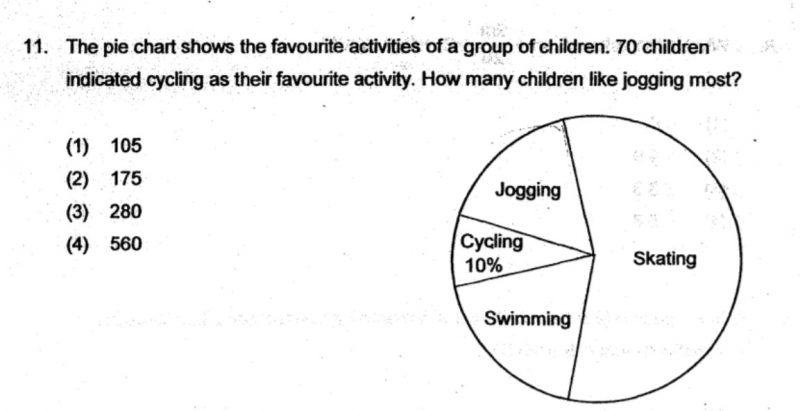# QuestionWe used protractor to measure the angle.

For cycling, it is 30 degrees

For Jogging, it is 60 degrees.

Really struggling to get 105 for jogging… Appreciate if you could kindly explain your thoughts.

Source: Singapore Chinese Girls’ Primary

A circle has a total of 360 degrees.

if

Jogging = 60 degrees

Cycling = 30 degrees

J+C = 90 degrees = 25% of 360 degrees.

C= 10%

J+C= 25%

J= 25% – 10% = 15%

Jogging = 700 x 0.15 = 105